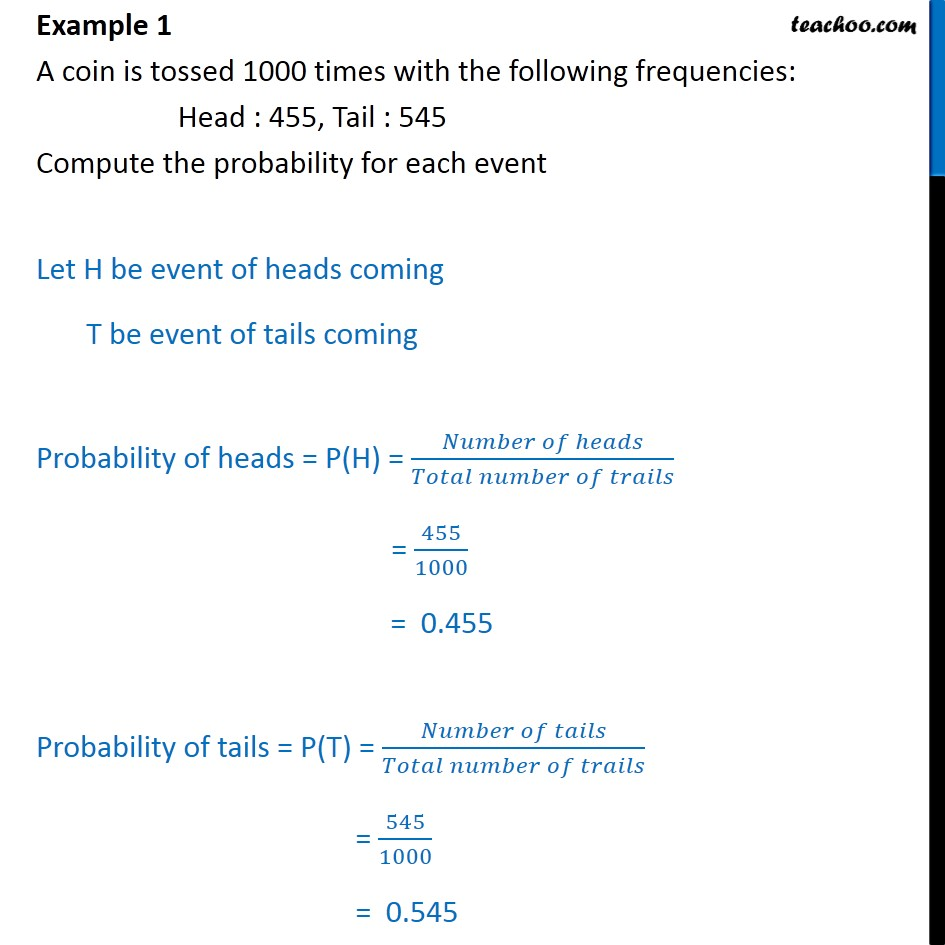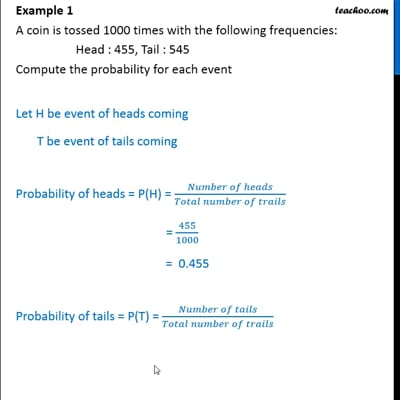Examples

Chapter 15 Class 9 Probability (Deleted)
Serial order wiseThis video is only available for Teachoo black users

Solve all your doubts with Teachoo Black (new monthly pack available now!)

### Transcript

Example 1 A coin is tossed 1000 times with the following frequencies: Head : 455, Tail : 545 Compute the probability for each event Let H be event of heads coming T be event of tails coming Probability of heads = P(H) = ( )/( ) = 455/1000 = 0.455 Probability of tails = P(T) = ( )/( ) = 545/1000 = 0.545